Maths-
General
Easy

Question

# Solve the system of equation by using elimination: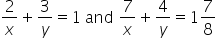Hint:

## The correct answer is: x = 8 ; y = 4

### Ans :- x = 8 ; y = 4Explanation :-Let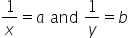now we get the equation in a and b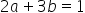— eq1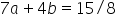— eq2Step 1 :- find value of b by eliminating aDo 2(eq2) - 7(eq1) to eliminate a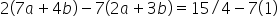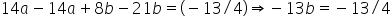∴ b = 1/4Step 2 :- find value of a by substituting b=1 in eq1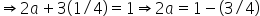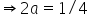∴ a = 1/8Step 3 :-  substitute a and b  inand find values of x and y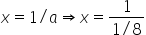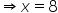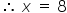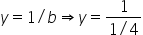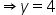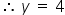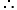x = 8 and y = 4 is the solution to the given pair of equations.#### With Turito Foundation.#### Get an Expert Advice From Turito.